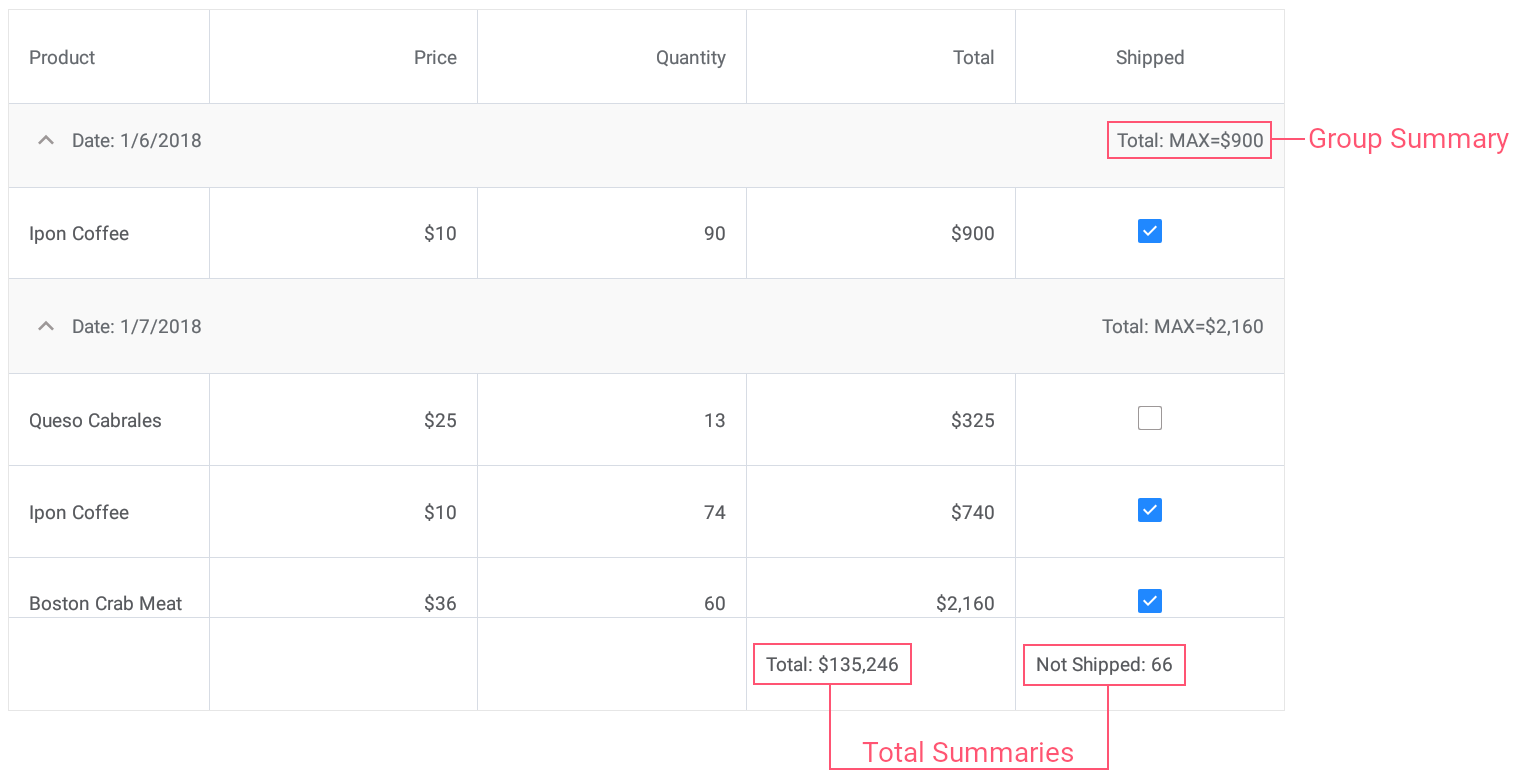21.1 (EAP/Beta)
20.2
20.1
19.2
19.1
1.1
The page you are viewing does not exist in version 1.1. This link will take you to the root page.

# DataGridView.CalculateCustomSummary Event

Allows you to specify a custom rule to calculate data summaries.

Namespace: DevExpress.XamarinForms.DataGrid

Assembly: DevExpress.XamarinForms.Grid.dll

## Declaration

``public event CustomSummaryEventHandler CalculateCustomSummary``

## Event Data

The CalculateCustomSummary event's data class is CustomSummaryEventArgs. The following properties provide information specific to this event:

Property Description
FieldName Gets the processed field name.
FieldValue Gets the processed field value.
GroupRowHandle
IsGroupSummary Gets whether a group summary value is being calculated.
IsTotalSummary Gets whether a total summary value is being calculated.
Item Gets an object that specifies a data source's item to which the grid's data row corresponds.
RowHandle Gets the grid's row handle.
SummaryProcess Gets a value that indicates the data summary calculation stage.
TotalValue Gets or sets the summary value.
TotalValueReady Gets or sets whether the Calculation stage of the custom summary calculation process should be skipped.

The event data class exposes the following methods:

Method Description
GetValue(String) Returns the value in the specified field.

## Remarks

Total and group summaries provide five predefined aggregate functions (Count, Max, Min, Sum and Average). To calculate a summary value using a custom rule, set the summary item's GridColumnSummary.Type property to SummaryType.Custom and handle the CalculateCustomSummary event.

The CalculateCustomSummary event fires for each data row involved in summary calculation. When calculating a total summary value, the event is raised for each data row. When calculating a group summary value, this event fires for each data row within a group. To enable you to perform any initialization and finalization, the event is also raised before and after processing rows.

The summary calculation consists of three stages.

• Initialization

The CalculateCustomSummary event is raised once and the CustomSummaryEventArgs.SummaryProcess property is set to Start . At this stage, you can initialize summary values (e.g., reset internal counters).

• Calculation

The CalculateCustomSummary event occurs multiple times, once for each data row in the grid or group. The SummaryProcess property is set to Calculate. At this stage, you should accumulate summaries.

• Finalization

The CalculateCustomSummary event is raised once and the SummaryProcess property is set to Finalize. At this point, calculate a final summary value and assign it to the event parameter's CustomSummaryEventArgs.TotalValue property.

To skip the Calculation stage and calculate a custom summary at the Initialization or Finalization stage, set the event parameter's CustomSummaryEventArgs.TotalValueReady property to true at the Initialization stage. This automatically skips the Calculation stage and the Finalization stage starts immediately.

## Examples

This example demonstrates how to use predefined aggregate functions and custom rule to calculate group and total summaries for grid columns.• Set the group summary to display the maximum Total value for each group of records.
• Set the total summary to calculate the sum of values in the Total column.
• Set the custom total summary to count the number of orders whose value in the Shipped column is false (to count orders that are not shipped).
``````<dxg:DataGridView x:Name="grid" ItemsSource="{Binding Orders}"
CalculateCustomSummary="OnCalculateCustomSummary">
<!-- ... -->
<dxg:DataGridView.GroupSummaries>
<dxg:GridColumnSummary FieldName="Total" Type="Max"/>
</dxg:DataGridView.GroupSummaries>

<dxg:DataGridView.TotalSummaries>
<dxg:GridColumnSummary FieldName="Total" Type="Sum"
DisplayFormat="Total: {0:C0}"/>
<dxg:GridColumnSummary FieldName="Shipped" Type="Custom"
DisplayFormat="Not Shipped: {0}"/>
</dxg:DataGridView.TotalSummaries>
</dxg:DataGridView>
``````
``````int count;
// ...

void OnCalculateCustomSummary(object sender, CustomSummaryEventArgs e) {
if (e.FieldName.ToString () == "Shipped")
if (e.IsTotalSummary){
if (e.SummaryProcess == CustomSummaryProcess.Start) {
count = 0;
}
if (e.SummaryProcess == CustomSummaryProcess.Calculate) {
if (!(bool)e.FieldValue)
count++;
e.TotalValue = count;
}
}
}
``````